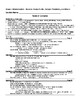# Grade 4 Math Student Study Guide and Sample ProblemsSubject
Resource Type
File Type
PDF (1 MB|45 pages)
Standards
\$15.00
• Product Description
• Standards

You can print out copies of this document for each student to use. Everything needed is contained within it. There are "short review sections" for each of the grade-level content standards, as assessed on the SBA Summative. This revision has added summarized Blueprints for the SBA Summative, the 2019-2020 IABs and ICA, a Student Data Tracker for IAB Data, and summarized information on how to access the student data in CAs CERS) system. The content is aligned with CAs mathematics content standards and the Common Core Standards.

There are sample problems, parallel in format to the SBA Summative, that students, in pairs, can work through to give them practice in solving real-life application problems.

Alternately, you could provide students with the information found in this document, and they can add it to their own interactive math journals during the year.

Compare two decimals to hundredths by reasoning about their size. Recognize that comparisons are valid only when the two decimals refer to the same whole. Record the results of comparisons with the symbols >, =, or <, and justify the conclusions, e.g., by using a visual model.
Use decimal notation for fractions with denominators 10 or 100. For example, rewrite 0.62 as 62/100; describe a length as 0.62 meters; locate 0.62 on a number line diagram.
Express a fraction with denominator 10 as an equivalent fraction with denominator 100, and use this technique to add two fractions with respective denominators 10 and 100. For example, express 3/10 as 30/100, and add 3/10 + 4/100 = 34/100.
Recognize a line of symmetry for a two-dimensional figure as a line across the figure such that the figure can be folded along the line into matching parts. Identify line-symmetric figures and draw lines of symmetry.
Classify two-dimensional figures based on the presence or absence of parallel or perpendicular lines, or the presence or absence of angles of a specified size. Recognize right triangles as a category, and identify right triangles.
Total Pages
45 pages